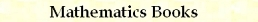ASCI Characters C/C++ Macros
by Stanislav Sýkora, Extra Byte, Via R.Sanzio 22C, Castano Primo, Italy 20022
in Stan's Library, Ed.S.Sykora, Vol.II. First release March 15, 2007.
Permalink via DOI:  10.3247/SL2Soft07.003
 PREVIOUS | NEXT | Code snippets | Software Books and Links Programming Section of Stan' LIBRARY | Stan's HUB
 This document is a part of a collection of macros. For introductory remarks, see the first part (General Purpose Macros). For a review of macro-syntax rules and related tips and tricks, see Writing Macros. For the typedefs CHAR and BYTE, click here.
 CATEGORY MACRO TESTS mIsCapLetter, mIsSmallLetter, mIsLetter, mIsWhitespace mIsDigit, mIsDecDigit, mIsHexDigit, mIsOctDigit, mIsBinDigit mIsChrOneOf2, mIsChrOneOf3, mIsChrOneOf4, mIsChrOneOf5, mIsChrOneOf6 CONVERSIONS mNoBit7 mUCaseChr, mLCaseChr mChr2Digit, mChr2HexDigit mDigit2Chr, mHexDigit2Chr, mHexDigit2chr, mLastBit2Chr
 ASCI characters tests Code: #define mIsLetter(ch) \     (!((((BYTE)(ch))<((BYTE)'A'))||((ch)>'z')||(((ch)>'Z')&&(((BYTE)(ch))<((BYTE)'a'))))) #define mIsCapLetter(ch) (((ch)>='A')&&(((BYTE)(ch))<=((BYTE)'Z'))) #define mIsSmallLetter(ch) (((ch)>='a')&&(((BYTE)(ch))<=((BYTE)'z'))) #define mIsWhitespace(ch) (((BYTE)(ch))<=((BYTE)0x20))   #define mIsDecDigit(ch) (((ch)>='0')&&(((BYTE)(ch))<=((BYTE)'9'))) #define mIsHexDigit(ch) (isDecDigit(ch)|| \     !((((BYTE)(ch))<((BYTE)'A'))||((ch)>'f')||((((BYTE)(ch))<((BYTE)'a'))&&((ch)>'F')))) #define mIsDigit(ch,hexok) (isDecDigit(ch)|| (hexok)? \     !((((BYTE)(ch))<((BYTE)'A'))||((ch)>'f')||((((BYTE)(ch))<((BYTE)'a'))&&((ch)>'F'))):0) #define mIsOctDigit(ch) (((ch)>='0')&&(((BYTE)(ch))<=((BYTE)'7'))) #define mIsBinDigit(ch) (((ch)=='0')||((ch)=='1'))   #define mIsChrOneOf2(ch,c1,c2) ((((ch)==c1)||((ch)==c2)) #define mIsChrOneOf3(ch,c1,c2,c3) (mIsChrOneOf2(ch,c1,c2)||((ch)==c3)) #define mIsChrOneOf4(ch,c1,c2,c3,c4) (mIsChrOneOf3(ch,c1,c2,c3)||((ch)==c4)) #define mIsChrOneOf5(ch,c1,c2,c3,c4,c5) (mIsChrOneOf4(ch,c1,c2,c3,c4)||((ch)==c5)) #define mIsChrOneOf6(ch,c1,c2,c3,c4,c5,c6) (mIsChrOneOf5(ch,c1,c2,c3,c4,c5)||((ch)==c6)) Arguments: ch, c1, c2, ... are values or expressions of the type char, CHAR or BYTE (or one which the compiler can automatically cast to one of these types). hexok is a flag of any type which can be either zero or nonzero. The return value is a Boolean type which can be tested for true (0) or false (1). These macros work also with wide, 16-bit characters (char_w) such as those of Unicode, provided one limits their use and meaning to the ASCI-compatible subset (bits 7-15 equal to 0). Notice the casting to (BYTE) of both sides of comparisons of the types < and ≤ This is necessary to force the compiler to do unsigned comparisons rather than a signed one (char is usually configured as signed short, and CHAR is defined in that way). Without the casting, bit 7 could play a havok; for example, the relation (ch)<'A' would be true for any character with bit 7 set. In situations where bit 7 is used as parity bit (e.g., communications of some types) or as a control bit (old Wordstar texts), you should first strip it off. In situation where it is meaningful (extended character sets), instead, the implementations presented here work correctly as they are. mIsLetter(ch) tests whether ch is a letter (A-Z or a-z). The implementation used here is a bit more efficient than the equivalent (mIsCapLetter(ch)||mIsSmallLetter()). mIsCapLetter(ch) tests whether ch is a capital letter (A-Z). mIsSmallLetter(ch) tests whether ch is a small letter (a-z). mIsWhitespace(ch) tests whether ch is a non-printing character (including space, tabulator, carriage-return, line-feed, etc). mIsDecDigit(ch) tests whether ch is one of the characters '0' - '9'. mIsHexDigit(ch) tests whether ch is one of the characters '0' - '9' or 'A'-'F' or 'a'-'f'. mIsDigit(ch,hexok) When hexok is true, tests whether ch is a hexadecimal digit; otherwise tests for decimal digit only. Use this macro only when hexok is defined dynamically at run time. Otherwise, use the more efficient macros mIsDecDigit or mIsHexDigit. mIsOctDigit(ch) tests whether ch is one of the characters '0' - '7'. mIsBinDigit(ch) tests whether ch is either '0' or '1'. mIsChrOneOf2(ch,c1,c2) mIsChrOneOf3(ch,c1,c2,c3) mIsChrOneOf4(ch,c1,c2,c3,c4) mIsChrOneOf5(ch,c1,c2,c3,c4,c5) mIsChrOneOf6(ch,c1,c2,c3,c4,c5,c6) tests whether ch is identical to one of the other characters.
 ASCI character conversions Code: #define mNoBit7(ch) ((ch)&0x7f)   #define mUCaseChr(ch) (isSmallLetter(ch)?((char)((ch)-32)):(ch)) #define mLCaseChr(ch) (isCapLetter(ch)?((char)((ch)+32)):(ch))   #define mChr2Digit(ch) ((ch)-'0') #define mChr2HexDigit(ch) \     ((((BYTE)(ch))<((BYTE)'A'))?mChr2Digit(ch):mUCaseChr(ch)-('A'-10) )   #define mDigit2Chr(k) ((char)((k)+'0')) #define mHexDigit2Chr(k) ((char)(((k)<10)?(k)+'0':(k)+('A'-10))) #define mHexDigit2chr(k) ((char)(((k)<10)?(k)+'0':(k)+('a'-10))) #define mLastBit2Chr(k) ((k)&1?'1':'0') Arguments: ch is a value or expression of the type char, CHAR or BYTE (or one which the compiler can automatically cast to one of these types). k is an integer-type value (or a type which the compiler can automatically cast to an integer). The return value depends upon the particular macro. These macros work also with wide, 16-bit characters (char_w) such as those of Unicode, provided one limits their use and meaning to the ASCI-compatible subset (bits 7-15 equal to 0). mNoBit7(ch) returns the character with bit 7 set to 0. In some contexts, bit 7 is used for parity-checking and has no semantic meaning. mUCaseChr(ch) When ch is a small letter, returns the corresponding capital letter; otherwise, returns it unmodified. mLCaseChr(ch) When ch is a capital letter, returns the corresponding small letter; otherwise, returns it unmodified. mChr2Digit(ch) returns the integer corresponding to ch (for example, character '3' gives the integer 3). No testing for the legality of the argument is done (use macro mIsDecDigit(ch) for that purpose). mChr2HexDigit(ch) returns the integer corresponding to ch (for example, characters '3' or 'C' give the integers 3 or 12, respectively). No testing for the legality of the argument is done (use macro mIsHexDigit(ch) for that purpose). mDigit2Chr(k) returns the decimal character '0'-'9'corresponding to the integer k, 0≤k<10. No testing for the legality of the argument is done; use mDigit2Chr((k)%10) when what you have in mind was the decimal modulus of k. mHexDigit2Chr(k) returns the hexadecimal character '0'-'9' or 'A'-'F' corresponding to the integer k, 0≤k<16. No testing for the legality of the argument is done; use mDigit2Chr((k)%16) when what you have in mind was the hexadecimal modulus of k. mHexDigit2chr(k) as above but the returned characters are '0'-'9' or 'a'-'f' (small letters). mLastBit2Chr(k) returns the binary character '0' or '1'corresponding to the least significant bit of k. Useful in conversions of integers into binary-representation strings.TOP | PREVIOUS | NEXT | Code snippets | Software Books and Links Programming Section of Stan' LIBRARY | Stan's HUB
 Copyright ©2007 Stanislav Sýkora    DOI: 10.3247/SL2Soft07.003 Designed by Stan Sýkora